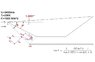# Solving for the Shockwave angle

• roldy
In summary, The engineer is having difficulty solving for the shock wave angle. He came across a pdf which explains the equation he needs, eq. (3.1). He solved for the shock wave angle using www.wolframalpha.com and found that it is 12 degrees at a Mach number of 1.4.f

#### roldy

I'm working on this calculation project and I need to solve for quantities at various stages of the system. I'm having difficulty in solving for the shock wave angle. I came across this pdf which explains everything that I'm attempting to do. It has the equation I need on page 2 eq. (3.1)

The knowns are as follows:
Mach=12
gamma=1.4
wedge deflection=9 degrees

http://www.aerostudents.com/files/aerodynamicsC/obliqueShockWaves.pdf" [Broken]

So I solved this using www.wolframalpha.com

The link where I actually input the function and the values for known variables is here:

http://www.wolframalpha.com/input/?i=solve+%28tan%289%29%3d2%2ftan%28beta%29*%2812^2*%28sin%28beta%29%29^2-1%29%2f%2812^2%281.4%2bcos%282*beta%29%29%2b2%29%2cbeta%29&s=46&incTime=true

The pdf states that for small values of the wedge deflection angle, two solutions will be present. So my question is...Which values of Beta from the wolframalpha.com site do I use?

Last edited by a moderator:

You have a 9 degree wedge angle, so where do you think the shock is going to be? You know it is a positive number. Does 88 degrees sound right here? (hint: that answer is quite ridiculous)

Voila.

This project includes a picture of the wedge and where the shock waves are, one created by the tip and also a reflected one due to the plate. Now with this picture, which answer makes sense. Note dimensions are given for the wedge but not for how much point im on the plate is extended past where the reflected wave meets the wedge.

#### Attachments

•shockwave copy.jpg
20.3 KB · Views: 656
Rule number 1, never use the picture as a guide for which answer to use. They are rarely drawn perfectly to scale.

So, let's go back to my original post. You got 2 positive answers out of your solution. You know the answer will be positive. Which of the two answers seems most plausible? Questions like these should be easy for an engineer to answer. You ought to be able to do a sanity check to see what makes sense and what doesn't. The two answers were 12 degrees and 88 degrees if I remember correctly. Does a small angle like 12 degrees make the most sense to you or does the large 88 degree angle that nearly approximates a bow shock make sense to you given the geometry of the problem?

That's what I'm having trouble deciding, which answer is most plausible. I've been doing some research and many procedures to this type of problem state that for small angle approximation, there will be two answers present. One for a strong shock and one for a weak shock. The weak shock case is something around 12* degrees and the strong shock is around 88 degrees. I see what you are saying with the bow shock approximation for 88 degrees. We haven't been taught just yet how to determine which shock angles to use when given 2 solutions. I would probably assume that's one of the underlying problems that our professor wants us to answer, even though he never stated it. If I had to choose an answer it would be the 12 degree shock angle. However, when I state this I have to give reasons why I decided this answer over the 88 degree shock angle solution. Thanks again for all your help and answering in a timely fashion.

I am sure you have seen pictures of shocks, right? What do they typically do as you increase Mach number. Don't worry about strong versus weak right now. In reality, only one will actually appear.

Yes, I have seen pictures of shocks. By observation I would assume that as the Mach number increases the shock wave angle decreases.

That would be a correct assumption. So with a sharp object at Mach 12, would you expect 12 degrees or the nearly normal 88 degrees?

I would expect 12 degrees. I guess this all makes sense now. Even though it should of clicked before, but this is new ground for me.

Right. Especially at high Mach numbers, you would expect the shock to be just barely above the surface like that. As you slow towards Mach 1, the two solutions will get closer together until at Mach 1 they merge and you get a normal shock.